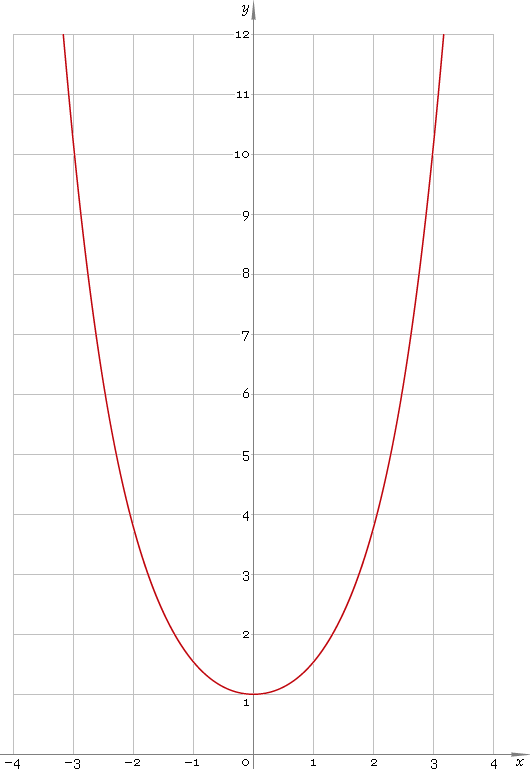The Art of Interface

# cosh or ch — hyperbolic cosine function

Category. Mathematics.

Abstract. Hyperbolic cosine: definition, plot, properties and identities.

## 1. Definition

Hyperbolic cosine is defined as

coshx ≡ (ex + ex) /2

## 2. Plot

Hyperbolic cosine is symmetric function defined everywhere on real axis. Its chain-line plot is depicted below — fig. 1.Fig. 1. Plot of the hyperbolic cosine function y = coshx.

Function codomain is range [1, +∞).

## 3. Identities

Base:

cosh2x − sinh2x = 1

Connection to exponential function:

sinhx + coshx = ex
coshx − sinhx = ex

By definition:

coshx ≡ 1 /sechx

Property of symmetry:

cosh−x = coshx

Half-argument:

cosh(x/2) = √[(coshx + 1) /2]
coshx = [1 + tanh2(x/2)] /[1 − tanh2(x/2)]

Doulbe argument:

cosh(2x) = sinh2x + cosh2x
cosh(2x) = 2 cosh2x − 1
cosh(2x) = 2 sinh2x + 1

Triple argument:

cosh(3x) = 4 cosh3x − 3 coshx

cosh(4x) = 8 cosh4x − 8 cosh2x + 4 = 8 cosh2x sinh2x + 1 = 8 sinh4x + 8 sinh2x + 1

Power reduction:

cosh2x = (cosh(2x) + 1) /2
cosh3x = (cosh(3x) + 3 coshx) /4
cosh4x = (cosh(4x) + 4 cosh(2x) + 3) /8
cosh5x = (cosh(5x) + 5 cosh(3x) + 10 coshx) /16

Sum and difference of arguments:

cosh(x + y) = coshx coshy + sinhx sinhy
cosh(xy) = coshx coshy − sinhx sinhy

Product-to-sum:

coshx coshy = [cosh(x + y) + cosh(xy)] /2
sinhx coshy = [sinh(x + y) + sinh(xy)] /2

Sum-to-product:

coshx + coshy = 2 cosh[(x + y) /2] cosh[(xy) /2]
coshx − coshy = 2 sinh[(x + y) /2] sinh[(xy) /2]

## 4. Support

Hyperbolic cosine function cosh or ch of the real argument is supported by free version of the Librow calculator.

Hyperbolic cosine function cosh or ch of the complex argument is supported by professional version of the Librow calculator.

## 5. How to use

To calculate hyperbolic cosine of the number:

``cosh(-1);``

To calculate hyperbolic cosine of the current result:

``cosh(rslt);``

To calculate hyperbolic cosine of the number x in memory:

``cosh(mem[x]);``